Current Postdocs and PhD students | Past Postdocs and PhD students

The past postdocs and PhD students at the Centre for Symmetry and Deformation are here listed with photos and research interests.  Click on a name to jump to research interests.

 Postdocs PhD students Michał Adamaszek  (2014-2016) Hiroshi Ando (2014-2015) Sara E. Arklint (2012-2017) Tobias Barthel (2016-2019) Samik Basu (2009-2011) Alexander Berglund (2009-2013) Kaj Börjeson (2017-2019) Chris Cave (2015-2018) Dustin Clausen (2013-2018) Tyrone Crisp (2012-2015) Christopher Davis (2013-2015) Dieter Degrijse  (2013-2016) Steven Deprez (2011-2014) Elden Elmanto (2018-2019) Dominic Enders (2013-2016) John Foley (2012-2015) Olivier Gabriel (2015-2017) Giovanni Gandini (2013-2015) Matthew Gelvin (2010-2012) Heiko Gimperlein (2010-2013) Magnus Goffeng (2015) Márton Hablicsek (2017-2019) Rune Haugseng (2015-2018) Markus Hausmann (2016-2019) Ellen Henke (2010-2013) Richard Hepworth (2009-2011) Gijsbert Heuts (2015-2017) Renee Hoekzema (2018-2019) Rune Johansen (2012-2015) Søren Knudby (2014) Job Kuit (2011-2014) Sander Kupers (2016-2017) Anssi Lahtinen (2010-2013) Cyril Levy (2009-2011) Frank H. Lutz (2013-2014) Ehud Meir (2012-2015) Kristian Moi (2014-2015) Johan Öinert (2010-2012) Irakli Patchkoria  (2013-2016) Dan Petersen (2014-2016) Maria Ramirez-Solano (2014) Oscar Randal-Williams (2010-2012) Leonel Robert (2010-2011) Simon Rose  (2015-2016) Beren Sanders (2014-2017) David Schrittesser (2014-2016) Farbod Shokrieh (2018-2019) Tatiana Shulman (2008-2011, 2013-2014) David Sprehn (2015-2018) Wolfgang Steimle (2013-2013) Gábor Szabó (2017-2018) Otgonbayar Uuye (2008-2011) Stefan Wagner (2012-2013) Guozhen Wang (2015-2016) Sinan Yalin (2016) Stella Anevski (2009-2013) Sara E. Arklint (2008-2011) Tarje Bargheer (2008-2011) Rasmus Bentmann (2010-2013) Kevin Aguyar Brix (2016-2019) Rasmus S. Bryder (2014-2017) Benjamin Böhme (2015-2018) Clarisson Canlubo (2014-2017) Martin S. Christensen (2014-2017) Thomas Danielsen (2009-2013) Emanuele Dotto (2009-2012) Chiara Esposito (2009-2011) James 'Jamie' Gabe (2012-2015) Matthias Grey (2012-2015) Casper Guldberg (2011-2015) Amalie Høgenhaven (2013-2017) Rune Johansen (2007-2011) Angela Klamt (2010-2013) Niek de Kleijn (2013-2016) Søren Knudby (2011-2014) Manuel Krannich (2016-2018) Tim de Laat (2010-2013) Isabelle Laude (2013-2017) Kang Li (2012-2015) Matias Lolk (2014-2017) Mauricio Gomez Lopez (2012-2015) Kristian Moi (2011-2014) George Napolitano (2008-2011) Espen Auseth Nielsen (2015-2018) Toke Nørgård-Sørensen (2010-2013) Kristian Olesen (2013-2016) Henrik D. Petersen (2009-2012) Valerio Proietti (2015-2018) Tomasz Prytula (2013-2017) Maria Ramirez-Solano (2009-2012) Sune P. Reeh (2011-2014) Daniela Egas Santander (2011-2014) Eduardo Scarparo (2014-2017) Adam Sørensen (2009-2012) Hannes Thiel (2009-2012) Massimiliano Ungheretti (2013-2016)

# Research interests

## PhD studentsMichał Adamaszek (PhD, Warwick 2012): My research is in combinatorial algebraic topology with connections to graph theory, applied topology and face enumeration. I am also interested in algorithmic and probabilistic aspects of these topics and often do computer experiments in my work.Hiroshi Ando (PhD, Kyoto 2012): I study structures of von Neumann algebras and some Polish groups related to them. Recently I have been working on ultraproducts of type III factors and some Borel equivalence relations related to unbounded self-adjoint operators on a separable Hilbert space.Sara E. Arklint (PhD, Copenhagen 2012): My research is concerned with classification of nonsimple (purely infinite) C*-algebras. So far I've been focusing on the classification functor filtered K-theory, and on the classification of Cuntz-Krieger algebras and graph algebras.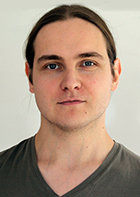Tobias Barthel (PhD, Harvard/Oxford 2014): I am interested in stable homotopy theory and its interactions with representation theory and algebraic geometry. In particular, much of my work is inspired by ideas from chromatic and transchromatic homotopy theory. More recently, I have also been thinking about various forms of (local) duality in algebra and topology.Samik Basu (PhD, Harvard 2009): I am interested in homotopy theory, particularly stable homotopy theory, and the obstruction theory of ring structures. My thesis was written about associative ring structures in generalised Thom spectra, and the computation of the Topological Hochschild Homology in certain examples.Alexander Berglund (PhD, Stockholm University, 2008): Algebraic topology and interactions with commutative algebra. Currently, my research interests revolve around operads, Koszul duality, and Hochschild cohomology of A-infinity algebras, with a view towards applications in String topology.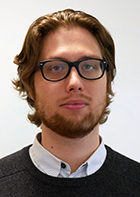Kaj Börjeson (PhD, Stockholm University 2017):I am interested in topology and algebra, especially topics connected to Koszul duality, rational homotopy theory, Hochschild cohomology, operads and BV-algebras.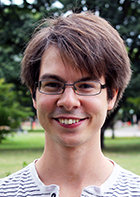Christopher Cave (PhD, Southampton 2015): My research is in coarse geometry which lies in the intersection of topology, geometry and operator algebras. My current interest in studying exact and non-exact groups and their consequences. I also have a lot of interaction with geometric group theory, in particular sequence of expanders graphs and their applications to geometric group theory.Dustin Clausen (PhD, MIT 2013): I am interested in connections between homotopy theory and number theory.Currently I'm studying a homotopy-theoretic approach to reciprocity laws. This approach is based on the equivalence between the category of finite free Z-modules, which can be thought of as number-theoretic, and the category of tori, which can be thought of as topological. Combined with the machinery of algebraic K-theory, this equivalence allows to use topological arguments to obtain number-theoretic results. Financed by Hesselholt's Niels Bohr Professorship.Tyrone Crisp (PhD, Penn State 2012): I study group representations, using tools from operator algebra and noncommutative geometry. At the moment I am particularly interested in reductive groups over local fields and rings, and in various kinds of induction/restriction constructions which relate representations of these groups to representations of their subgroups.Christopher Davis (PhD, MIT 2009): My research is primarily in the areas of number theory and algebraic geometry, especially p-adic cohomology. Through Witt vectors and the de Rham-Witt complex, my research is also related to algebraic topology. Financed by Hesselholt's Niels Bohr Professorship.Dieter Degrijse (Phd, Leuven 2013): My research interests include geometric group theory, algebraic topology and homological finiteness conditions.Steven Deprez (PhD, Leuven 2011): My research centers on the study of type II1 factors. There are many constructions for type II1 factors, but it is very hard to decide if two, a priori different, constructions give the same type II1 factor. For example, every ICC group G gives rise to a type II1 factor LG. But all amenable ICC groups G give the same type II1 factor LG, which we call the hyperfinite type II1 factor. For that reason, various invariants have been introduced, for example the fundamental group (which is not related to the fundamental group of a topological space) and the outer automorphism group. These invariants are usually very hard to compute, but Sorin Popa's deformation/rigidity theory allows us to compute these invariants in specific cases. Often, type II1 factors are constructed from groups or from actions of groups on probability spaces. Deformation/rigidity theory uses measurable and geometric properties of these groups and actions. For that reason I am also interested in measurable and geometric group theory.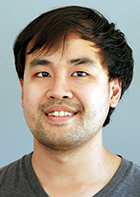Elden Elmanto (PhD, Northwestern 2018): My research interesest is in algebraic geometry and its interactions with homotopy theory. This occurs mainly through Morel-Veovodsky's motivic homotopy theory, the theory of motives, and algebraic K-theory.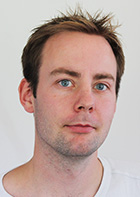Dominic Enders (Phd, Westfälische Wilhelms-Universität Münster, 2013): My main research interests are operator algebras, in particular the structure theory and classification of C*-algebras. More precisely, I am interested in semiprojectivity (or, more generally, perturbation questions), dimension theories for C*-algebras, classification via K-theory and the interplay between those topics.John D. Foley (PhD, UCSD 2012): My research combines algebraic topology and geometric group theory to study infinite (or infinite dimensional) groups. I am especially interested in the possible extension of homotopy Lie groups as developed in the theory of p-compact and p-local compact groups to homotopy Kac-Moody groups. More broadly, I am interested in how n-types generalize groups as higher symmetries and how homotopy can be used to study small models combinatorially.Olivier Gabriel (PhD, Paris 7 2011): My research interests center on operator algebras and noncommutative geometry. I work with spectral triples, quantum groups, K-theory and cyclic cohomology. I am especially interested in ergodic actions on C*-algebras.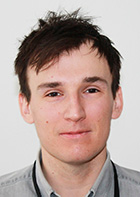Giovanni Gandini (PhD, Southampton 2011): I work in geometric group theory, often using tools from group cohomology. I am particularly interested in (homological) finiteness properties of groups. Recently I also took an interest in the isomorphism conjectures.Matthew Gelvin (PhD, MIT 2010): I work in the intersection of algebraic topology and finite group theory, studying fusion systems and their classifying spaces.  My graduate thesis describes group actions from a fusion- and p-local finite group-theoretic perspective; I hope to continue in this vein by exploring the representation theory of fusion system and their connections with modular representation theory.Heiko Gimperlein (PhD, Hannover 2010): My research centers around geometric analysis on noncompact or singular manifolds and includes applications to representation theory and numerical analysis. Particular topics are pseudodifferential operators on singular spaces, K-theory of the corresponding operator algebras, propagation of singularities for the wave equation, analytic methods for Lie groups and their representations as well as theoretical numerical analysis of nonconvex variational problem.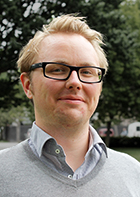Magnus Goffeng (Phd, University of Gothenburg 2015): My research evolves around the more analytic aspects of non-commutative geometry. It is mainly concerned with problems from index theory and spectral theory.Márton Hablicsek (PhD, University of Wisconsin-Madison 2014): My research is in algebraic geometry with focus on Derived Algebraic Geometry and Combinatorial Algebraic Geometry.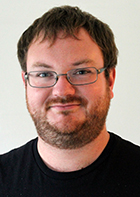Rune Haugseng (Phd, Massachusetts Institute of Technology 2013):  I am interested in algebraic topology and higher category theory, and their connections with other areas such as (derived) geometry and (topological) quantum field theory.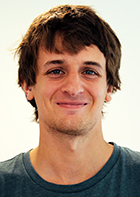Markus Hausmann (Phd, University of Bonn, 2016): My research is in algebraic topology, in particular different forms of equivariant homotopy theory. At the moment I am studying global equivariant spectra and try to apply them to problems in equivariant and non-equivariant topology.Ellen Henke (PhD, Birmingham 2010): My research focuses on studying saturated fusion systems. These are categories satisfying important features of fusion in finite groups. They have been studied by algebraic topologists, modular representation theorists and recently also by local group theorists. Many concepts and results from finite group theory have been translated into the language of fusion systems. An important long term goal appears to be the classification of all simple saturated fusion systems, at least for the prime 2. Achieving this goal will certainly require a huge amount of work, and with my research I hope to contribute to that. The main project I am working on at the moment aims to classify certain fusion systems that I call minimal. Minimal fusion systems can be seen as analogs of Thompson's N-groups whose classification set a pattern for the classification of finite simple groups. Moreover, they appear to play a significant role, since every non-solvable fusion system has a section which is minimal.Richard Hepworth (PhD, Edinburgh 2005): I work in the field of Algebraic Topology, and in particular in String Topology. This was introduced almost 10 years ago by Chas and Sullivan, and it shows how to extract interesting algebra from a manifold by thinking about the loops, or strings, in that manifold. I recently proved a result that completely describes the algebraic quantities when the manifold is a Lie group, and I am currently hoping to extend this in several directions.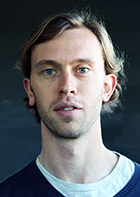Gijsbert Heuts (PhD, Harvard 2015): I am interested in studying unstable homotopy theory using methods from Goodwillie calculus and chromatic homotopy theory. I also think about higher category theory and its applications to these topics.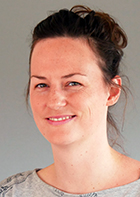Renee Hoekzema (PhD, Oxford, 2018): My mathematics research concerns algebraic and geometric topology, particularly in the study of manifolds, cobordism categories and topological quantum field theories. I am also interested in mathematical (palaeo-)biological questions as well as mathematical physics, particularly relativity and quantum gravity.Rune Johansen (PhD, Copenhagen 2011): My research focuses on the interplay between symbolic dynamics and operator algebras, and I use computer programs to do experimental investigations of such mathematical structures in the search for input to formulate conjectures, theorems, and proofs. Currently, I am primarily working on experimental investigations of automorphisms of graph algebras and isomorphisms of Leavitt path algebras. I am also interested in the flow equivalence classification of sofic shifts with a special focus on renewal systems and beta-shifts. Additionally, I am involved in a project concerning colouring problems for LEGO-buildings.Søren Knudby (PhD, Copenhagen 2014): My main interest is the study of von Neumann algebras and relations to group theory. In particular, I study approximation properties for groups and von Neumann algebras to see how they relate and complement each other. A classic example is that of amenability which for a group may be formulated as an approximation property and is reflected as hyperfiniteness of the group von Neumann algebra.Job Kuit (PhD, Utrecht 2011): My research centers around integral geometry and harmonic analysis on symmetric spaces. I am particularly interested in horospherical transforms. Together with Erik van den Ban (Utrecht University) and Henrik Schlichtkrull (University of Copenhagen) I am currently working towards a notion of cusp form for reductive symmetric spaces. We hope to get a better understanding of the Plancherel decomposition for these spaces by using cusp forms.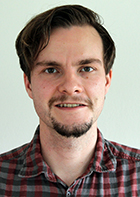Alexander Kupers (PhD, Stanford 2016): My research is in applications of algebraic topology to algebra and geometry. In particular, I am interested in the homology groups and homotopy groups of spaces of automorphisms of various objects.Anssi Lahtinen (PhD, Stanford 2010): I am an algebraic topologist with interests in string topology, parametrized homotopy theory and field theories. I am currently especially interested in the string topology of classifying spaces, which is a field studying the homology groups of the free loop space of the classifying space of a compact Lie group. As discovered by Chataur and Menichi, these homology groups enjoy a rich algebraic structure, as they are the value of the circle in a Homological Conformal Field Theory, a field theory where the operations are parametrized by homology classes of spaces of cobordisms. Jointly with Richard Hepworth, I am working on a novel construction of these field theories that will improve on Chataur and Menichi's original construction in a number of ways.Cyril Levy (PhD, ENS-Lyon 2009): I am interested in the construction of spectral triples for Dirac operators on manifolds with boundary and applications to the spectral action of Chamseddine-Connes. We recently obtained, with Bruno Iochum and Dmitri Vassilevich, a regular spectral triple for a rather general geometric setting which includes the skew-symmetric torsion and the chiral bag conditions on the boundary. It turns out that theta=0 is a critical point of the associated spectral action in any dimension and at all orders of the expansion.  The first four leading terms of the action have been computed for vanishing chiral parameter (theta=0), and torsion-dependent terms have been identified.Frank H. Lutz (PhD, Berlin 1999): My main field of research is experimental topology. I have been involved in various projects on constructing extremal or otherwise interesting triangulations of manifolds. With Jesper Møller I work on colorings of simplicial complexes and manifolds. Together with Bruno Benedetti and Karim Adiprasito I have initiated and is developing random discrete Morse theory. Mimi Tsuruga and FL recently combinatorialized the Akbulut-Kirby handle body description of the Cappell-Shaneson spheres. The resulting triangulations are used, in collaboration with Konstantin Mischaikow and Vidit Nanda, as non-trivial examples for testing the homology software CHomP. With Menachem Lazar, Robert MacPherson, Jeremy Mason and David Srolovitz I work on the topological microstructure analysis of metals and steel.Ehud Meir (PhD, Technion 2010): I work in cohomology of groups and in Hopf algebras, as well as in some related areas. My Phd dealt with some questions relating the cohomology of a group to that of a finite index subgroup. I later studied some questions which relates finite dimensional Hopf algebras to properties of the field over which they are defined. For example, if H is a finite dimension semisimple Hopf algebras (over a field F of characteristic zero), we can ask what are the possible simple quotients of H (I have proved that up to Brauer equivalence we get everything), or if H is already defined over the ring of integers of F (in a joint work with Juan Cuadra, we have proved that it is not always the case). I have also studied fusion categories, which can be viewed as a generalization of the representation categories of Hopf algebras.Johan Öinert (PhD, Lund 2009): My research is concerned with the ideal structure of non-commutative rings, in particular graded rings such as generalized crossed products and skew group rings. I am also interested in the interplay between topological dynamical systems and various types of algebraic constructions such as C*-crossed product algebras.Irakli Patchkoria (PhD, Bonn 2013): My research interests mainly lie in stable homotopy theory and homological algebra. I am especially interested in equivariant stable homotopy theory.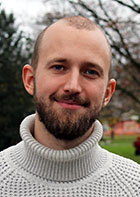Dan Petersen (PhD, KTH Stockholm 2013): I am interested in the topology and cohomology of algebraic varieties, particularly moduli spaces.Maria Ramirez-Solano (PhD, Copenhagen 2013): I work in the research area of operator algebras and tilings. I am currently working with Uffe Haagerup on the experimental side of the investigation of the Thompson group F. Part of my PhD thesis involved computer experiments while investigating a non-standard hierarchical tiling.Oscar Randal-Williams (DPhil, Oxford 2009): I am interested in studying "moduli spaces of manifolds", both in the sense of diffeomorphism groups of manifolds, which are very interesting yet opaque, and in the sense of the spaces representing cobordism theory, which are far more computable. The Madsen-Weiss theorem identifies spaces of these two flavours in dimension 2: a large part of my research, joint with Søren Galatius, is towards generalising this result to higher-dimensional manifolds. The other aspect of my research is focused on applying results centered around the Madsen-Weiss theorem to answer cohomological questions about spaces related to moduli spaces of curves, for example to study the universal Picard variety, or moduli spaces of r-spin curves.Leonel Robert (PhD, Toronto 2006): My field of research is the classification and structure of C*-algebras. In its broadest formulation, the classification problem asks "What is the *right* invariant that classifies the* right* class of C*-algebras?" Although there may not be a unique answer to this question, various structural properties of the C*-algebra arise naturally when looking for the right class: Z-stability, finite nuclear dimension, the dichotomy of purely infinite vs stably finite, approximation by nice" subalgebras, etc. On the side of the classifying invariants, the most standard ones are K-theory (filtered, with coefficients), the cone of traces, and more recently, the Cuntz semigroup.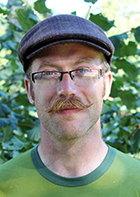Simon Rose (PhD, University of British Columbia 2012) I study the enumerative geometry of hyperelliptic curves and surfaces, and in particular their connections with number theory and physics. Lately I have taken an interest in tropical geometry and how it fits into this picture.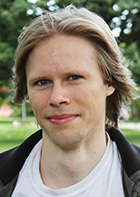Beren Sanders (PhD, UCLA 2014) My primary research interests lie in the theory and applications of triangulated categories, especially tensor triangular geometry and examples arising in stable homotopy theory, modular representation theory, algebraic geometry, and noncommutative topology. Other interests include equivariant homotopy theory, motivic homotopy theory, higher category theory, and the representation theory of groups and associative algebras.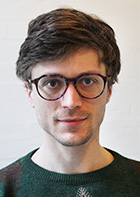David Schrittesser (PhD, Vienna 2010): My research is in set theory, where I have worked on forcing axioms, large cardinals, and descriptive set theory. I work on problems in descriptive set theory, but also, for example, on forcing and some questions connected to the automorphism group of the measure algebra. I also hope to be able to apply descriptive set theoretic methods in other fields, especially operator algebra.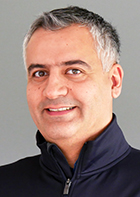Farbod Shokrieh: I am interested in non-Archimedean analytic and tropical geometry, combinatorics, algebraic and arithmetic geometry.Tatiana Shulman (PhD, Moscow 2006): I am working in Operator Algebras. Since each commutative C*-algebra (operator algebra) is the algebra of all continuous functions on some (locally) compact space, one can try to generalize topological notions to the category of all (not necessary commutative) C*-algebras. In particular there is a theory of noncommutative absolute retracts and absolute neighborhood retracts which I am very ineterested in.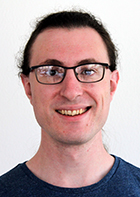David Sprehn (PhD, University of Washington 2015):  I work in group cohomology.  I am studying the modular cohomology of the general linear groups over finite fields, and of other finite groups of Lie type.  More generally, I am interested in group actions and characteristic classes.Wolfgang Steimle (PhD, Münster 2010): My research focuses on the interplay between the topology of manifolds and algebraic K-theory. More specifically, I am interested in the fibering problem ("Is a given map between manifolds homotopic to a fiber bundle projection?"), in metrics of positive scalar curvature ("Are there exotic families of such metrics on a given manifold?"), and on the Bökstedt-Madsen map that relates to cobordism category to Waldhausen's K-theory. Financed by Hesselholt's Niels Bohr Professorship.Gábor Szabó (PhD, University of Münster 2015): My research focuses on the fine structure of simple C*-algebras and the classification of group actions on these objects. I am also interested in the structure of crossed products, in particular regarding the interplay between C*-algebras and topological dynamics.Otgonbayar Uuye (PhD, Penn State 2008): I  study K-theory and KK-theory of operator algebras and index theoretic  problems in NCG using cyclic cohomology.I did my PhD with Nigel Higson at Penn State on the local index theorem in noncommutative  geometry; I studied index characters of K-homology classes over noncommutative spaces, in particular multiplicativity properties, meromorphic continuation of regularized traces, asymptotic expansions  of heat kernels etc.Stefan Wagner (PhD, Darmstadt 2011): My research is mainly concerned with translating the geometric ideas and concepts of the theory of fibre bundles to Noncommutative Geometry. In particular, I am interested in the noncommutative geometry of principal bundles (and hence as well in noncommutative generalizations of coverings). I am also interested in finding "geometric" invariants for noncommutative spaces - currently I am working on a noncommutative version of the fundamental group for C*-algebras.Guozhen Wang (PhD MIT 2015): My research interest is homotopy theory, especially those computational aspects. My current interest is computing stable and unstable homotopy groups of spheres, using techniques from chromatic theory and Goodwillie calculus.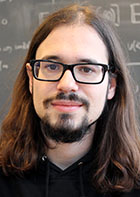Sinan Yalin (PhD Lille 1 2013): My research focuses on interactions between homotopy theory of algebraic structures and various topics in topology, geometry and mathematical physics. For this, I use methods coming from homotopy theory, homotopical algebra, higher category theory and derived geometry.

## PhD studentsStella Anevski (advisor: R. Nest): My research interests are arithmetic geometry and non-commutative geometry. In particular, I am interested in characteristic classes of Hermitian vector bundles. My scientific advisor is professor Ryszard Nest.Sara E. Arklint (advisor: S. Eilers): I am working within the field of noncommutative geometry. In particular I am interested in invariants and lifting problems of C*-algebras. My thesis is concerned with the question of whether filtered K-theory can be used as a complete invariant for purely infinite, nuclear, separable C*-algebras with a finite ideal lattice. Filtered K-theory consists for a given C*-algebra of the K-theory of all the subquotients of the C*-algebra together with some natural transformations between these groups. The main result of the thesis is that there exist finite ideal lattices where classification fails in general but where filtered K-theory is a complete invariant if one restricts to graph algebras or real rank zero C*-algebras (depending on the lattice). The thesis also describes the range of filtered K-theory for graph algebras with some finite ideal lattices.Tarje Bargheer (advisor: N. Wahl): My project is initiated by string topology, providing algebraic structure -- in a suitable homotopy theoretic sense -- on the free mapping space of a circle into a manifold. I have during my Ph.D been working on constructing a gadget -- the cleavage operad. The cleavage operad generalize string topology by providing algebraic structure on the free mapping space of a euclidean embedded manifold N into another manifold M. For the case of euclidean spheres, I have shown that the algebraic structure doesn't change much from the circle case. However; deforming -- and knotting -- the embedding of N has quite drastic consequences on the complexity of the algebraic structure. Examining these consequences is ongoing research. Potentially it is related to von Neumann Algebras via Vaughan Jones' planar algebras, passing through Khovanov homology.Rasmus Bentmann (advisors: S. Eilers & R. Nest): An important tool in the classification theory of non-simple C*-algebras is a version of Kasparov's KK-theory for C*-algebras over non-Hausdorff spaces due to Eberhard Kirchberg. I am interested in finding K-theoretic invariants computing this equivariant KK-theory by means of a universal coefficient theorem. This problem depends sensitively on the acting space in question. Currently I am working out the construction of an adjunction comparing the resulting triangulated categories for certain pairs of finite spaces.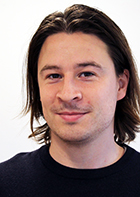Kevin Aguyar Brix (advisor: S. Eilers): I am interested in the interrelation between topological dynamical systems and their induced operator algebras. More precisely, I study to which degree relations such as flow equivalence, orbit equivalence or conjugacy is reflected and remembered in the corresponding C*-algebras along with its diagonal.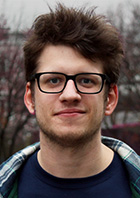Rasmus Sylvester Bryder (advisors: M. Musat & M. Rørdam): My research interests lie in the field of operator algebra. At the moment I'm looking into the relationship between groups and their reduced group C*-algebras, with an overall goal being to determine how different group C*-algebras can be from other classes of C*-algebras.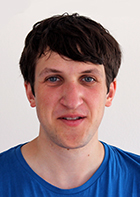Benjamin Böhme (advisor: J. Grodal): I am interested in equivariant algebraic topology, especially in the various homotopy theories of G-spaces and G-spectra. Very similar to classical ring theory, idempotents in the Burnside ring A(G) yield splittings of G-spectra. However, not much is known about the "algebraic" properties of these decompositions: In what way do (E-infinity) ring structures of the pieces relate to that of the original spectrum? What information do we obtain when restricting attention to localizations or completions, one prime at a time? The goal of my PhD project is to find answers to some of these questions.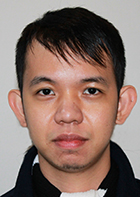Clarisson Canlubo (advisior: R. Nest): My mathematical interests center in noncommutative geometry and all its related areas. At the moment, I am trying to generalize algebraic topological invariants like homotopy groups and monodromy actions in NCG. I am also interested in quantum group theory, K-theory, homological algebra, algebraic topology, and Galois theory. During my spare time, I study algebraic geometry and mirror symmetry. In particular, I am learning derive algebraic geometry.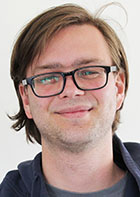Martin Søndergaard Christensen (advisor: M. Rørdam): I am primarily interested in the theory of operator algebra, in particular the classification of C*-algebras. Currently I am studying certain divisibilty and comparibility properties of C*-algebras A, usually expressed in terms of the Cuntz semigroup Cu(A), in an effort to understand the relationship between these properties and structural properties of the associated central sequence algebra.Thomas Danielsen (advisor: H. Schlichtkrull): I'm working within the field of harmonic analysis on Lie groups and homogenous spaces. For any homogenous space G/H there is a so-called Plancherel decomposition of the regular representation of G on L^2(G/H). If G/H is a symmetric space, this Plancherel decomposition was determined by H. Schlichtkrull and E. van den Ban. At the moment I'm trying to find the Plancherel decomposition of the (non-symmetric) space SL(2,R)xSL(2,R)xSL(2,R)/SL(2,R) hoping that it can give some insight on how to generalize the Plancherel formula to more general non-symmetric homogenous spaces.Emanuele Dotto (advisor: I. Madsen): My interests are divided in two subjects. In the beginning of my thesis I worked on h-principles with boundary conditions. I used simplicial categories techniques coming from the study of the weak homotopy type of cobordism categories to determine if the scanning map for a topological sheaf restricted and corestricted to elements having a prescribed behavior on the boundary remains a weak equivalence. Now I am working on real algebraic K-theory. This is a spectrum with involution associated to a ring (spectrum) with an antistructure. I am interest in the fiber of the map in K-theory induced by a well behaving ring map. The methods I am using (trace maps) compare this fiber with the fiber of the map induced in a gadget called real topological cyclic homology, where computations are easier. The case without involution has been solved by McCarthy and Dundas, and I am trying to generalize this result in this equivariant setting.Chiara Esposito (advisor: R. Nest): My research interest are symmetries and deformation quantization. In particular, my thesis is concerned with quantum momentum maps as a deformation of the classical one. I am focused on the general definition of classical momentum map related to the Poisson action of a Poisson Lie group and so I have started working with Poisson structures and their quantization given by quantum groups.James Gabe (advisors: S. Eilers & R. Nest): My main interests are operator algebra, more specifically classification of C*-algebras using K-theoretic data. At the moment I am trying to classify certain (graph) C*-algebras with infinitely many ideals using K-theory invariants.Matthias Grey (advisor: I. Madsen): I am interested in automorphisms of highly connected high dimensional manifolds.Casper Guldberg (advisors: A. Berglund & N. Wahl): The overall goal of my research is to study spaces of homotopy automorphisms. I have just started to look at this from the point of rational homotopy theory, and for a class of particularly nice spaces there is an explicit chain complex from which we in principle can compute the rational homotopy groups of the homotopy automorphisms. However, even in some very simple cases the computations become rather complicated.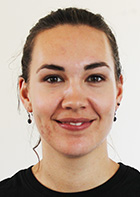Amalie Høgenhaven (advisor: L. Hesselholt): My research interest lie in algebraic K-theory and homotopy theory in general. I’m especially interested in topological cyclic homology and equivalent stable homotopy theory. My PhD-project is concerned with calculations in the newly defined real algebraic K-theory and the associated real topological cyclic homology. Financed by Hesselholt's Niels Bohr Professorship.Rune Johansen (advisor: S. Eilers): I work with C*-algebras associated to symbolic dynamical systems, and I am particularly interested in the classification of sofic shift spaces with respect to flow equivalence. Another part of my thesis concerns a longstanding conjecture for renewal sytems. So far, my main results concern the structure of various standard presentations of sofic shift spaces and the ideal structure of the corresponding C*-algebras.Angela Klamt (advisor: N. Wahl): I am working in algebraic topology. In particular I am interested in Frobenius algebras and homological conformal field theories. A homological conformal field theory (HCFT) is a symmetric monoidal functor to the category of graded vector spaces from a category whose morphisms are given by the homology of mapping class groups of surfaces. Costello shows that the Hochschild homology of "A-infinity Frobenius algebras" gives examples of homological conformal field theories. Another example is the homology of the free loop space of a manifold. It appears that many of the operations given by a HCFT are trivial in many cases. In the current state of my PhD I investigate reasons given by the algebraic structure to generalize these vanishing results. Another question I want to investigate is when the action of the HCFT comes from a chain level action. The above mentioned vanishing results might help to solve this question.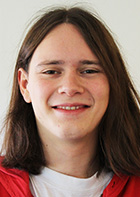Niek de Kleijn (advisor: R. Nest): My research centers around formal deformation quantization, specifically obtaining algebraic index theorems. When one considers the smooth functions on some Poisson manifold there is the obvious commutative algebra structure. Through deformation quantization one can deform this algebra structure into a non- commutative one, such that the commutator of two functions is given by the Poisson bracket. This idea of quantizing the smooth functions arises naturally in passing from classical mechanics to quantum mechanics. On the other hand one can obtain from such deformed algebras index theorems a la Atiyah-Singer. Currently I am studying explicit deformations in the case of a symplectic manifold with an action of an infinite group and corresponding explicit formulations of algebraic index theorems.Søren Knudby (advisor: U. Haagerup): My main interest is the study of von Neumann algebras and relations to group theory. In particular, I study approximation properties for groups and von Neumann algebras to see how they relate and complement each other. A classic example is that of amenability which for a group may be formulated as an approximation property and is reflected as hyperfiniteness of the group von Neumann algebra.Manuel Krannich (advisor: N. Wahl): Currently, my research revolves around homological stability of topological groups. Besides that, I am interested in bordism categories and moduli spaces of manifolds—mainly initiated through my master’s thesis.Tim de Laat (advisors: U. Haagerup & M. Musat): The theory of operator spaces provides powerful tools for the study of operator algebras. My research is on the interface between these two areas. To begin with, I will work on a number of problems related to Grothendieck inequalities in the noncommutative setting. I also aim to use the theory of completely bounded maps to answer questions about approximation properties of operator algebras, in the spirit of Grothendieck's programme, which also relates to my interest in Lie groups. For example, I want to investigate completely bounded Fourier multipliers on a noncompact Lie group and applications to approximation properties for  the group von Neumann algebra and the reduced group C*-algebra associated to discrete subgroups of the given Lie group.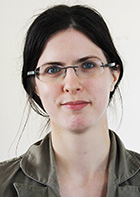Isabelle Laude (advisor J. Grodal): My primary interest is the connections between group theory and homotopy theory, particularly in the case of p-local finite groups and localities. I study mapping spaces with target the classifying space for a p-local finite group to understand the homotopical analogs of group theoretical concepts such as elements of p-power order and centralizers for the classifying space of a p-local finite group.Kang Li (advisors: U. Haagerup & R. Nest): My research is mainly concerned with Approximation Theory in C*-Algebras, K-Theory/E-Theory for C*-Algebras, Deformation/Rigidity Theory for von Neumann Algebras and Geometric Group Theory. Currently, I study the exactness for locally compact groups and its applications to the injectivity of the assembly map in the Baum-Connes conjecture.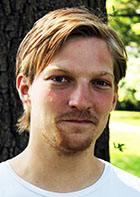Matias Lolk (advisor: M. Rørdam): My research focuses on a class of C*-algebras related to so-called separated graphs, introduced by Pere Ara and Ruy Exel. While the theory offers many interesting examples that deserve to be studied on their own, the main question is if the construction gives rise to (nuclear) real rank zero C*-algebras with exotic non-stable K-theories. So far, my work has centered on characterising nuclearity and exactness and describing the ideal strucure in terms of the graph - in particular characterising simplicity. In the near future, I will also start studying obstructions to real rank zero.Mauricio Gomez Lopez (advisor: E. K. Pedersen): My research interests lie in the in the intersection of piecewise linear topology and moduli spaces of manifolds in the style of Galatius and Randal-Williams. More specifically, in the articles The Homotopy type of the cobordism category' and Monoids of moduli spaces of manifolds' Galatius, Madsen, Tillmann, Weiss and Randal-Williams have described the homotopy type of the smooth embedded cobordism category and the main goal in the first part of my PhD is to prove an analgous result in the piecewise linear setting.Kristian Moi (advisor: I. Madsen): My research centres around algebraic K-theory. In particular, for a ring A with an (anti)-involution it makes sense to talk about hermitian (bilinear) forms on A-modules. With certain finiteness and non-degeneracy assumptions such modules can be studied using algebraic K-theory; this is called hermitian K-theory. Ordinary algebraic K-theory of a ring studies modules over the ring, and this turns out to be closely related to the topological Hochschild homology and topological cyclic homology of the ring. Now I am working to understand the relationship between the hermitian K-theory of a ring with involution and its topological hochschild homology and topological cyclic homology.George Napolitano (advisor: B. Durhuus): My research area is mathematical physics, in particular I'm interested in discretized quantum gravity models. In this field planar maps are of particular interest, since they play the role of two-dimensional discretized space-time manifolds. As planar maps can be put, under suitable conditions, in a bijective  correspondence with planar trees, I'm currently studying ensemble of trees, in particular the so called well-labelled trees. For time being I'm trying to construct a probability measure,defined by a local weight function, on sets of infinite trees.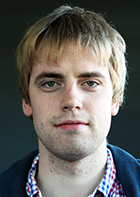Espen Auseth Nielsen (advisor: N. Wahl): I am interested in homotopy theory and homotopical algebra. I am studying the Hochschild homology of En-algebras.Toke Nørgård-Sørensen (advisor: J. Grodal): In my PhD I plan to study p-compact groups (p is some prime). A lot of the theory of p-compact groups is analogous to theory of compact Lie groups -- also from any connected compact Lie group one can construct a p-compact group. I plan to look a the representation theory of p-compact groups.Kristian Knudsen Olesen (advisors: U. Haagerup & M. Musat): My research interests are in the theory of von Neumann algebras and its relation to geometric group theory, through objects such as the group von Neumann algebra, and the reduced group C*-algebra.  Of particular interest are approximation properties for groups and C*-algebras.Henrik D. Petersen (advisor: R. Nest): My main interests are L^2 invariants for groups (and other objects)- in particular trying to understand higher L^2-Betti numbers and extract information from the non-vanishing of these.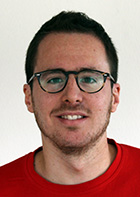Valerio Proietti (advisor: R. Nest):  In Paris I started working on my Master's thesis on the gap labeling conjecture. This is a problem which originates in solid-state physics and is expressed in the language of noncommutative geometry. The temporary sketch of my PhD thesis is based on the study of K-theoretic and cohomological invariants for C*-algebras arising from dynamical systems associated to actions of finite-rank abelian groups. These dynamical systems might emerge from physical contexts, therefore the interplay between modern physics and noncommutative geometry will play a role.Tomasz Prytula (advisor: J. Møller): I will be studying connections between combinatorial and homotopy theoretical properties of Davis-Januszkiewicz spaces.Maria Ramirez-Solano (advisor: E. Christensen): My research interests lie within the borderlines of differential geometry and non-commutative geometry. I am currently researching the use of spectral triples in C*-algebras and other methods from non-commutative geometry to investigate certain features of fractal geometry, and the structure of tilings of the plane. The natural non-smoothness of fractals could for example be overcome by employing spectral triples to measure distances and volumes of such objects. Furthermore I plan to investigate the spectrum of quasiperiodic Hamiltonians and if we can apply the theory of quasicrystals to circle packings. There are visible analogies between aperiodic tilings and circle packings in the plane, sphere or hyperbolic disk, and I would also like to investigate the possibilities to study equivalence classes of circle packings as non-commutative spaces as it has been done in connection with the study of quasicrystals and the quantum Hall effect.Sune Precht Reeh (advisor: Jesper Grodal): My main area of interest is algebraic topology and its applications to group theory. I am currently working with saturated fusion systems and how they relate to the Burnside ring of the underlying p-group, in particular those elements of the Burnside ring which are “stable” with respect to the fusion system. This relates to work by Ragnarsson and Stancu showing that saturated fusion systems are classified by the so-called characteristic idempotents in the double Burnside ring of the underlying p-group.Daniela Egas Santander (advisor: N. Wahl): My interests lie in graph complexes and the moduli space of Riemann surfaces.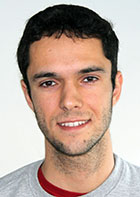Eduardo Scarparo (advisor: M. Rørdam): I am interested in C*-algebras, paradoxical conditions for groups and group actions on C*-algebras.Adam Sørensen (advisor: S. Eilers): I have two main research interests: Semiprojectivity and graph algebras. I am interested in the closure properties of the class of semiprojective C*-algebras, and semiprojectivity of Real C*-algebras with respect to matrix algebras. The latter can be view as the study of almost commuting real matrices.For graph algebras, my main interest is which properties of the algebras can be 'seen' on the graph. In particular what it means for two graphs to have stably isomorphic algebras.Hannes Thiel (advisor: M. Rørdam): I study the classifiability of C*-algebras, in particular: What is the right notion of a "classifiable" C*-algebra in the stably finite case? It is known that the Elliott classification conjecture will only hold assuming some regularity properties, and there are various of these (Z-stability, strict comparison of positive elements, finite dimension) I study the relation between these properties.Massimiliano Ungheretti (advisor: N. Wahl): The objective of my project is to describe field theories related to cyclic homology.